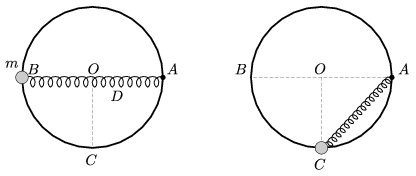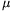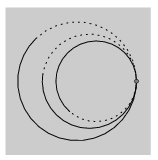Mathematical and Physical Journal
for High Schools
Issued by the MATFUND Foundation
 Already signed up? New to KöMaL?

# KöMaL Problems in Physics, January 2012

Show/hide problems of signs:## Problems with sign 'M'

Deadline expired on February 10, 2012.

M. 320. Drill (or in any other way make) a small hole to the bottom of a 1.5 litre plastic bottle, and fill the bottle with water to a height of H. Measure how the time T during which the water flows out of the water depends on the diameter of the hole and on the height of the water H. According to theoretical reasons T is proportional to some power of H. According to the measured data what is this exponent?

(6 pont)

statistics## Problems with sign 'P'

Deadline expired on February 10, 2012.

P. 4402. We have 8 Christmas-tree bulbs, rated at 14 V and 3 W, and among old lumbers bulbs rated at 110 V and 10 W, 24 W, 40 W and 60 W are found. Which one should be connected in series with the 8 Christmas-tree bulbs, also connected in series, in order that all the nine bulbs operate properly, when they are connected to a 220 V voltage supply?

(3 pont)

solution (in Hungarian), statistics

P. 4403. At three different locations of a scout camp there are three troops, each at a distance of 1 km from another. Electric bells are to be installed in order that any of the troops can give signal to another troop. Draw the circuit diagram. How much wire is needed? a) At each location there is a battery, a bell and two press-buttons. b) Instead of the three batteries only one is available.

(4 pont)

solution (in Hungarian), statistics

P. 4404. A floating wooden-cube of density 600 kg/m3, and of edge 10 cm, is pushed down very slowly right below the surface of the water a) in a lake; b) in a vessel the area of the bottom of which is 3 dm2. Plot the force as a function of the displacement of the cube, and calculate the work done in both cases.

(4 pont)

solution (in Hungarian), statistics

P. 4405. A small object of mass m=0.4 kg is threaded onto a vertical ring of radius r=30 cm, and of centre O. The object can move frictionless along the ring. One end of a spring of spring constant D=16 N/m, and of unstretched length 30 cm is attached to the small object and its other end is fixed to point A on the horizontal diameter of the path. Find the speed of the object when it passes the lowest point of the path C, if it is released from rest at point B. In this case what is the force exerted by the object on the ring, and what is the direction of its acceleration at point C?(4 pont)

solution (in Hungarian), statistics

P. 4406. A sound source is at rest and emits a sound of frequency f0=250 Hz for some time. The driver of the car which is moving towards the source at a speed of v, detect this sound the frequency of which is f'=263 Hz, and he hears the sound exactly as long as he covers a distance of s=82 m, along a straight line. a) What is the speed of the car? b) For how long did the sound source emit the sound?

(5 pont)

solution (in Hungarian), statistics

P. 4407. In district heating pipes there are two metal wires embedded in the insulating foam surrounding the pipe. The wires run parallel to the axis of the pipe (see photo on back cover). What is the purpose of these wires, when are they needed?

(4 pont)

solution (in Hungarian), statistics

P. 4408. Somewhere in the desert there is a cylinder shaped vessel containing gas, and at the top of the vessel there is an easily moveable piston which separates the gas inside and the ambient air without any leakage. Early in the morning when the temperature is 0 oC the bottom part of the piston is at a height of 2 m, measured from the bottom of the cylinder. During the military attack later in the morning a bullet accidently made a hole in the cylinder at a height of 2.2 m. What fraction of the mass of the gas leaks out if during the day the temperature can increase even to 60 oC?

(4 pont)

solution (in Hungarian), statistics

P. 4409. The capacitor of capacitance C=12.5F in an ideal resonant circuit is charged to U1=400 V, and then the circuit is closed. The inductance of the coil is 0.5 H. a) Give the current as a function of time. b) In what time intervals will the energy of the capacitor be maximum?

(4 pont)

solution (in Hungarian), statistics

P. 4410. On a sunny day, when there was no cloud on the sky, someone observed an interesting phenomenon. There was a plastic triangle shaped ruler next to the window on the table, and observing the ruler a pattern of coloured band was seen on it, similar to that of formed on the surface of oil on wet asphalt. a) Could this phenomena be the result of the interference of the reflected light-beams from the bottom and the top of the ruler, as in case of the oil-spot? b) If not, what might the explanation be?

(5 pont)

solution (in Hungarian), statistics

P. 4411. In a sample initially there are equal number of atoms of two radioactive isotopes A and B. Three days later there are 3 times as much nucleides of isotope A than of isotope B, the halflife of which is 1.5 days. What is the half-life of isotope A?

(4 pont)

solution (in Hungarian), statistics

P. 4412. Experiments carried out in cloud chambers make it possible to observe the path of charged particles moving in uniform magnetic field. Is it possible that during a charged particle decays to two other particles the circular paths which touch each other, and which are shown in the figure can be seen in the cloud chamber? (The declaration of the particles are negligible on the examined part of the motion.)(6 pont)

solution (in Hungarian), statistics